## Sum( and Seq( commands on your calculator

Can you find the sum of the following series?$\displaystyle \sum_{i=1}^{15}\dfrac{2n+1}{3n-2}$

This is neither an arithmetic nor a geometric series, so you don’t have a formula for it. This would be a tedious problem to do by hand. Fortunately, your graphing calculator can do these problems quickly and efficiently.

There are two functions you need to use on your calculator. The seq( command creates a sequence of terms based on a rule that you give. The sum( command adds together the terms in a sequence. Both functions are found on the LIST menu on your calculator. The seq( command is on the OPS submenu and the sum( command is on the MATH submenu.

To sum a series, you combine the two commands. If you have the new operating system on your calculator, it will prompt you for the entries when you select the seq( command. If you have the old operating system, you need to know the syntax for the command. The syntax for the series above is:

sum(seq((2x + 1)/(3x – 2),x,1,15))

Note that the seq( command has four parameters in the parentheses. From left to right, these are 1) the rule for the nth term of the sequence; 2) the variable name; 3) the first value of the variable; and 4) the final value of the variable. Now all you need to do is type this in to your calculator and let it do the crunching:

## The “parent” functions

Do you know your parent functions? I’m surprised every year when some of my students don’t know how to graph some of the elementary functions they’ve learned in class. Your chances for success in precalc and calculus are significantly better if you memorize the shapes of these ten functions. No excuses, no shortcuts. Just learn them.

y = xn (Power functions)

y = |x| (absolute value function)

y = ⌊x⌋ (Greatest integer function)

y = √x

y = 1/x

y= bx (exponential function – growth and decay)

y = log x

y = sin x and y = cos x

y = tan x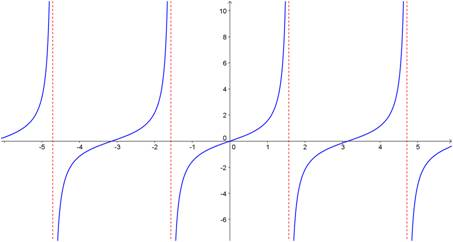## Factoring sums and differences of fifth powers (and higher!) when the power is odd

Occasionally, you are required to factor a polynomial that is in the form of a sum or difference of two power terms (i.e., x– yn). In my last post, I showed you a simple procedure you can learn to help you factor the binomial if the value of the power is 4 or greater and the power is even. [If you are reading this post, you should already be familiar with factoring the difference of two squares and the difference of two cubes. Check this website for posts on those procedures.] In this post, I show you a procedure (more complicated than the others, I admit) that you can use to factor these binomials when the power is odd.

First, let’s see how this works on some examples:

Do you see the pattern? When factoring a– bn or a+ bn (for n odd), there is always a linear term a ± b, where the plus or minus sign is the same as in the original binomial. The remaining factor is a little more complicated, but it does have a simple pattern. Each term consists of the first term in the binomial (with its exponent decreasing from n – 1 to 0) and the second term in the binomial with its exponent increasing from 0 to n – 1. In the first example above, the x term decreased from x4 to x0. The 2 term increased from 20 to 24. If you started with the difference of two powers, all of the signs in the second factor are plus signs. If you started with the sum of the two powers, the first sign in the second factor is a minus sign and the signs alternate after that.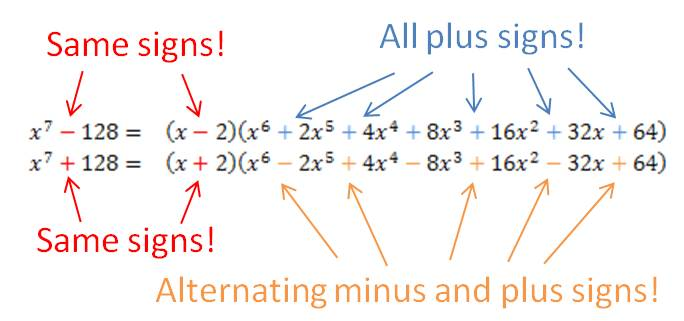## Factoring differences of fourth powers (and higher!) when the power is even

Occasionally, you are required to factor a polynomial that is in the form of a difference of two power terms (i.e., xn – yn). There is a simple procedure you can learn to help you factor the polynomial if the value of the power is 4 or greater and the power is even. [If you are reading this post, you should already be familiar with factoring the difference of two squares and the difference of two cubes. Check this website for posts on those procedures.]

First, let’s see how this works on some examples:

You can see that whenever the power is even, you treat the binomial as the difference of two squares and find its factors. If the factors are still the sum and difference of perfect squares, you repeat the process with the term that is the difference of two squares. If the factors are now the sum and difference of perfect cubes, you follow the method for factoring the sum or difference of two cubes. If the powers are sums and differences of odd powers higher than 3, there is another procedure to factor them. You can find another post on this website that shows that procedure.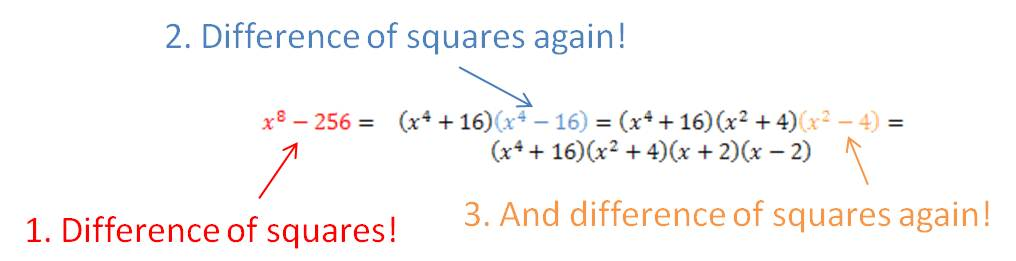## Simplifying “3-stack” and “4-stack” fractions

I had a physics student a number of years ago who worked a complicated problem and ended up with the following (I’ve changed the actual numbers to make our work here easier to follow):$\dfrac{(2)(3)}{4}=\dfrac{(5)(6)(7)B}{(8)(9)}$

Of course, his next step was to solve for B. It should be clear to you, as it was to him, that he needed to multiply both sides by 8 and 9 and divide by 5, 6 and 7. That is what he did. But this is how he wrote the solution:$B= \dfrac{9 \cdot \left( \dfrac{ \left(\dfrac{ 8 \cdot \left( \dfrac{(2)(3)}{4} \right)}{5} \right)}{6} \right)}{7}$

His expression was totally correct, and he found the correct value of B, but he made the problem so much harder than he needed to. He created a fraction with five different stacks in it and he needed all those parentheses to keep track of which number was a numerator and which was a denominator.

This is an extreme case of what I see so many students do: they create “3-stack” and “4-stack” fractions all the time when they are simplifying problems. Let’s look at two examples. I’ll solve them with “bad” solutions and then with much smarter solutions (I don’t simplify the answers here because I want to focus on the first step of the solution):

Example 1. Solve:$3x= \dfrac{2}{5}$$x= \dfrac{\dfrac{2}{5}}{3}$

Smarter solution:$x= \dfrac{2}{5 \cdot 3}$

Example 2. Solve:$\dfrac{4}{7}x=\dfrac{2}{5}$$x= \dfrac{\dfrac{2}{5}}{\dfrac{4}{7}}$

Smarter solution:$x= \dfrac{2 \cdot 7}{5 \cdot 4}$

You can see that the smarter solutions are simpler to read and easier to simplify.

It is easy to train yourself to write answers as “2-stack” fractions if you remember one simple rule:

Respect the vinculum.

Um, respect the what?!?

The vinculum. When you write a fraction, the horizontal line that separates the numerator from the denominator is called the vinculum. No one ever talks about it, but it’s a very powerful symbol. It tells you to multiply by every number that is above it and divide by every number that is below it. And it’s as easy as that. So when you are simplifying expressions like the ones in the two examples above or the more complicated example at the beginning of this post, all you need to do is to put numbers that are multiplied above the vinculum and numbers that are divided below the vinculum. And what happens if you are multiplying or diving by a fraction? Then put the numerator on top and the denominator on the bottom if you are multiplying. Flip the fraction over first if you’re dividing (as I did in Example 2). When you do this, you will end up with a 2-stack fraction that can be easily evaluated. Let’s look at the original problem again:$\dfrac{(2)(3)}{4}=\dfrac{(5)(6)(7)B}{(8)(9)}$

To solve for B, you will multiply by 8 and 9, so they go on top. And you will divide by 5, 6 and 7, so they go on the bottom. In one step, you’ve solved for B as follows:$B= \dfrac{2 \cdot 3 \cdot 8 \cdot 9}{5 \cdot 6 \cdot 7}$

Compare that to the monstrosity at the beginning. So much easier!

P.S. You don’t really have to remember the name “vinculum”. Most people don’t know what the line is called and don’t care. Chances are good your math teacher doesn’t even know the term. To make things even more bizarre, when you write a fraction with a slash instead of a horizontal line like this—2/3 – the slash is called a “virgule”.  Most people don’t know that one and don’t care about it either. All you have to remember is that the horizontal line in a fraction tells you to multiply on top and divide on the bottom.

## A mnemonic for remembering values on the unit circle

In an earlier post, I told you that you must memorize the values on the unit circle if you want to be great at trigonometry. So let’s say that you have taken my advice, but on a test you have a big brain cramp and you forget the values. Here’s a trick that will help you fill in the values in the first quadrant. The five angles in the first quadrant are 0°, 30°, 45°, 60°, and 90°. And the sines of these angles just happen to be:$\underline{ \qquad \theta \qquad \sin \theta \qquad \qquad \qquad}$$0 \textdegree \qquad \; \; 0 \qquad =\dfrac{\sqrt{0}}{2}$$30 \textdegree \qquad \dfrac{1}{2} \qquad = \dfrac{\sqrt{1}}{2}$$45 \textdegree \qquad \dfrac{\sqrt{2}}{2} \quad = \dfrac{\sqrt{2}}{2}$$60 \textdegree \qquad \dfrac{\sqrt{3}}{2} \quad = \dfrac{\sqrt{3}}{2}$$90 \textdegree \qquad 1 \qquad = \dfrac{\sqrt{4}}{2}$

Do you see the pattern in the last column? The sines increase from$\dfrac{\sqrt{0}}{2}$ to$\dfrac{\sqrt{4}}{2}$

That’s an easy pattern to remember even during the worst brain cramp. Why does it work? It’s just a coincidence. But if you use this pattern, you can fill in the sine values in the first quadrant. Then the cosine values are the same in reverse order. Once you’ve completed the first quadrant, you can fill in the rest of the unit circle by using reference angles. Now you have a completed unit circle!

## Using the reciprocal key on your calculator

Your graphing calculator has a reciprocal key on it, designated as$x^{-1}$. It’s the easiest way to find the reciprocal of a number on your screen. Just press the key, and then Enter.

The reciprocal key is also a great way to do calculations of resistors in parallel or capacitors in series. For example, find the equivalent resistance of a circuit that has a 10 Ω and a 15 Ω resistor in parallel. Simply enter it this way into the calculator to find the answer is 6 Ω.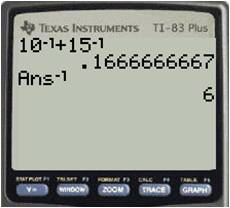## Factoring the difference of two squares

You will spend a lot of time in algebra (and courses beyond) factoring polynomials into linear and quadratic terms. There are some special polynomials that occur so frequently that you should recognize them on sight so that you know the method for factoring them. The most common is the binomial of the form$a^2-b^2$. This is called the difference of two squares because both terms are perfect squares and you are subtracting (finding the difference between) the two terms.

Some examples of this binomial are$x^2-4$,$9x^4-16y^2$ and$121y^2z^2-25w^4a^6b^{12}$. Note that in each case, both terms are perfect squares.

It is very easy to factor these expressions—you can do it by inspection. The rule is simple and you must memorize it:$a^2-b^2=(a+b)(a-b)$. Once you determine that an expression is the difference of two squares, you can write out its factors immediately. Let’s see how it works with the three examples given above:$x^2-4=(x+2)(x-2)$$9x^4-16y^2=(3x^2+4y)(3x^2-4y)$$121y^2z^2-25w^4a^6b^{12}=(11yz+5w^2a^3b^6)(11yz-5w^2a^3b^6)$

It’s really that easy once you learn the format. Of course it doesn’t matter what order you write the two factors, so$x^2-4=(x-2)(x+2)$ is also correct.

One other important point: this factoring rule works in reverse, too! If you have to distribute (multiply out) two factors and you see they are in the form of$(a+b)(a-b)$, you should recognize that this gives the difference of two squares:$a^2-b^2$. You can do this immediately, without multiplying out every term. Learning this formula will save you lots of time.

## Graphing rational functions—4. Drawing the graph

In previous posts, I described the steps you follow to analyze a rational function. If you follow these steps, you can be a rational function superstar too. In this post, I put all the pieces together to show how you use the information you’ve obtained to plot the graph. [For details on how to execute the various steps, please see other posts on this website.]

Example: Graph the following rational function.$f(x)=\dfrac{2x^3-16x^2+38x-24}{x^3-4x^2+x+6}$

Solution: We break this into many steps.

1. Find the$y$-intercept:$f(0)=\dfrac{-24}{6}=-4$

2. Fully factor the function:$f(x)=\dfrac{2x^3-16x^2+38x-24}{x^3-4x^2+x+6}=\dfrac{2(x-1)(x-3)(x-4)}{(x+1)(x-2)(x-3)}$

3. Find the vertical asymptotes, if any:

The vertical asymptotes are at$x=-1$ and$x=2$.

4.Find the$x$-intercept(s), if any:$f(x)=0$ @$x=1$ and$x=4$

5. Find the hole(s), if any:

There is a hole at the point$(3,-1)$

6. Find the horizontal or oblique asymptote, if any, or characterize the end behavior:

The horizontal asymptote is at$y=2$

7. Place all of this information on the graph:8. Use all the information you have plotted to complete the graph of the function: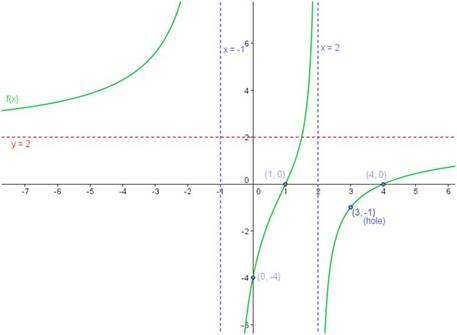## Graphing rational functions—3. Finding horizontal or oblique asymptotes

In a previous post, I listed the steps you need to follow to analyze and graph a rational function. In this post, I show you how to find the horizontal or oblique asymptotes, if they exist. If there are no horizontal or oblique asymptotes, then you can determine the end behavior of the function. [Check other posts on this website for other steps in analyzing and graphing a rational function.]

The horizontal and oblique asymptotes, if they exist, tell you the end behavior of the function. That is, they describe what the function is doing as$x$ goes to ±∞. In order to determine the end behavior, examine the degrees of the polynomials in the numerator and denominator. There are four possible cases:

Case 1: The degree of the denominator is greater than the degree of the numerator.

When the denominator has the higher degree, the denominator grows much faster than the numerator as$x$ gets very large. Like all fractions, as the denominator gets very large, the fraction gets very small and approaches zero. So the asymptote is$y=0$.

Example 1: Find the end behavior for the following rational function:$f(x)=\dfrac{x^2+3x+2}{x^3+4x^2+x-6}$

Solution: Because the degree of the numerator is$2$ and the degree of the denominator is$3$, this function has a horizontal asymptote$y=0$.

Case 2: The degree of the denominator is equal to the degree of the numerator.

When the degrees of the numerator and denominator are the same, the function approaches a finite non-zero number. This number is the ratio of the leading coefficients of the two polynomials and this is the value of the horizontal asymptote.

Example 2: Find the end behavior for the following rational function:$f(x)=\dfrac{6x^2+7x-4}{5x^2-4x-6}$

Solution: Because the degree of the numerator and denominator are the same, this function has a horizontal asymptote. Divide the leading coefficients ($6$ and$5$) and the horizontal asymptote is$y=6/5$.

Case 3: The degree of the numerator is exactly one higher than the degree of the denominator.

In this case, there is an oblique asymptote (called a slant asymptote in some textbooks). To find the asymptote, divide the denominator into the numerator using long division. The quotient is the oblique asymptote. (Ignore the remainder; it has no effect on the asymptote.)

Example 3: Find the end behavior for the following rational function:$f(x)=\dfrac{x^3+4x^2+x-6}{x^2+3x+2}$

Here, the numerator (degree 3) is exactly one higher than the denominator (degree 2). To find the asymptote, perform the following long division: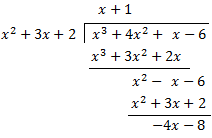The quotient is the oblique asymptote:$y=x+1$

Case 4: The degree of the numerator is more than one higher than the degree of the denominator.

In this case, there is no horizontal or oblique asymptote. Instead, the end behavior of the function is the same as the end behavior of a polynomial whose degree is the same as the difference between the degrees of the numerator and the denominator. For example, if the numerator is degree$5$ and the denominator is degree$2$, the rational function will look like a cubic function as$x$ approaches ±∞.

Blue Taste Theme created by Jabox